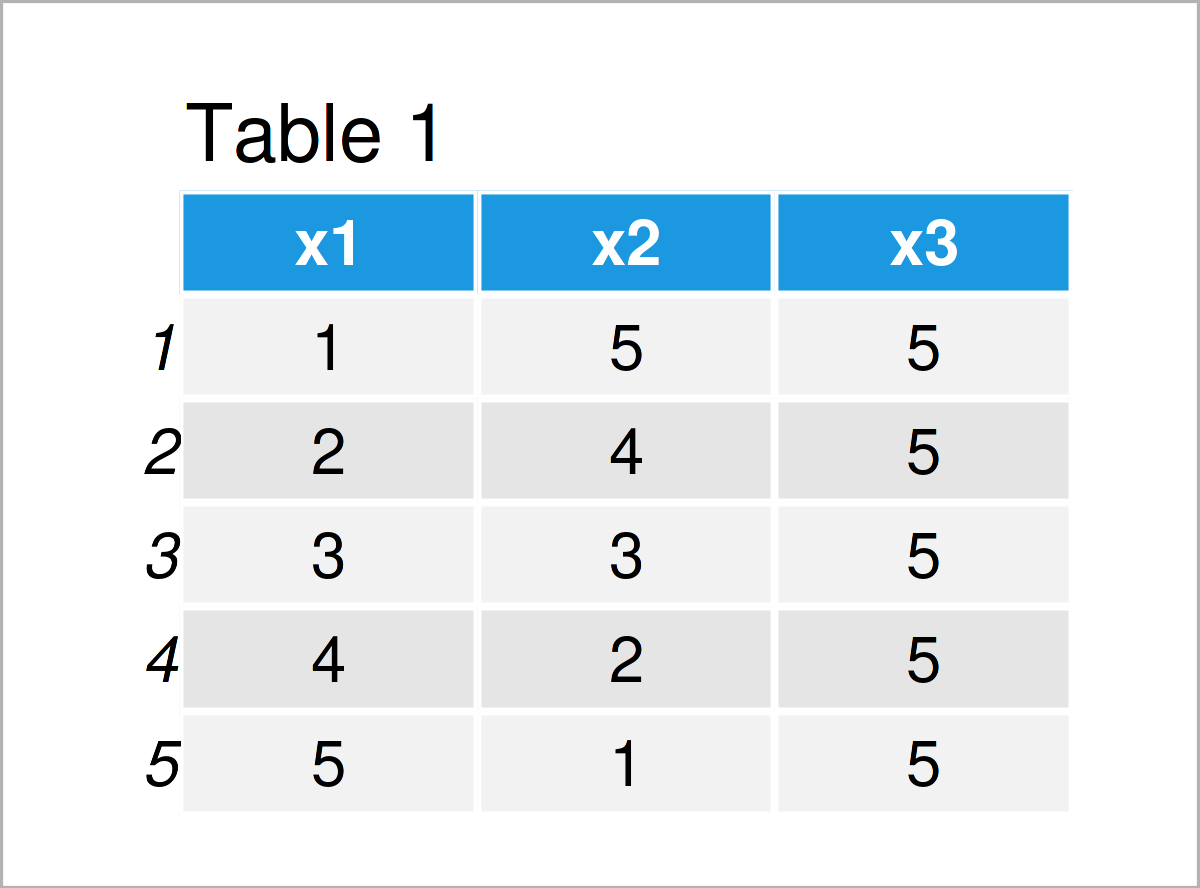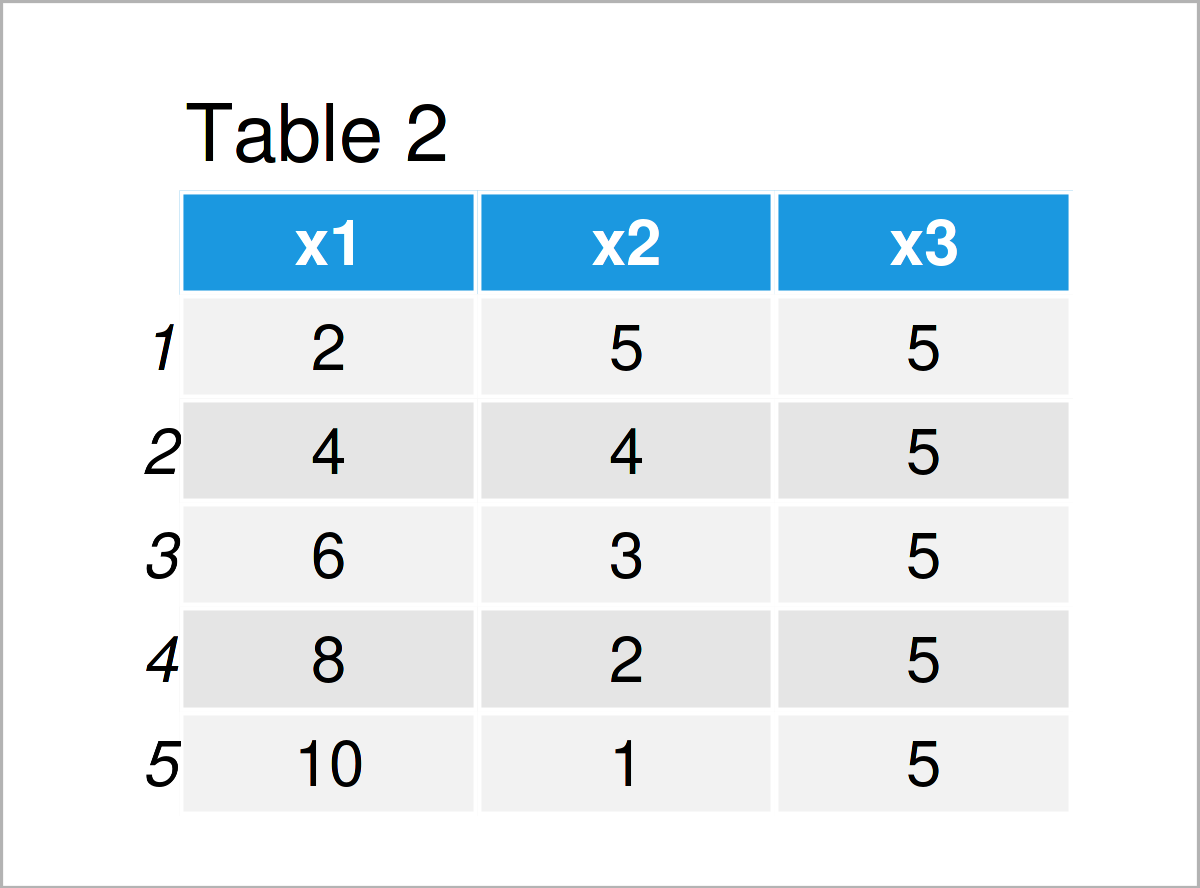# Replace Entire Data Frame Column in R (2 Examples)

In this tutorial, I’ll show how to exchange a whole column of a data frame in the R programming language.

The table of content looks as follows:

Let’s take a look at some R codes in action:

## Creation of Example Data

We’ll use the following data as basement for this R programming tutorial:

```data <- data.frame(x1 = 1:5,    # Create example data
x2 = 5:1,
x3 = 5)
data                            # Print example data```Table 1 shows the structure of our example data – It consists of five rows and three columns.

## Example 1: Transform Values in Column

The R programming syntax below demonstrates how to substitute an entire data frame variable with a transformed version of this variable.

More precisely, let’s assume that we want to replace the numerical values in the variable x1 with those values multiplied by two.

Then, we can use the \$ operator and the assignment arrow as shown below:

```data\$x1 <- data\$x1 * 2          # Transform values in column
data                            # Print updated data```As revealed in Table 2, the previous R programming code has managed to create an updated version of our input data frame where the column x1 was multiplied by two.

## Example 2: Replace Column by Entirely New Values

Example 2 demonstrates how to replace an entire column by absolutely new values that are not related to the values currently stored in this column.

For this, we can use the R code below:

```data\$x1 <- letters[1:5]         # Replace column by new values
data                            # Print updated data```By executing the previous R code we have created Table 3, i.e. a data frame with new values in the column x1.

## Video & Further Resources

Do you need further info on the R codes of this tutorial? Then you could have a look at the following video on my YouTube channel. In the video, I demonstrate the R programming codes of this post:

Furthermore, you might want to have a look at the related posts on this website.

In this R tutorial you have learned how to replace an entire variable of a data frame. Please let me know in the comments, in case you have any additional comments and/or questions.

Please note that we have used the \$ operator to replace data frame columns in the present tutorial. However, the R programming language provides further operators for the subsetting and exchange of data that have not been discussed on this page. For an overview of useful operators, you may have a look here.